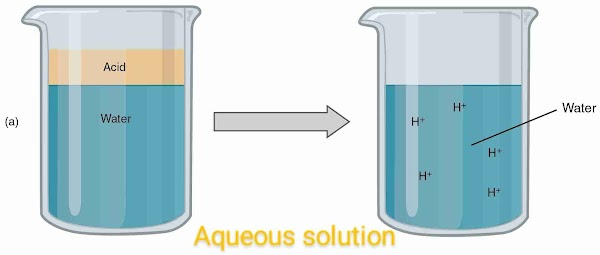## why does an aqueous solution of an acid conduct electricity

### why does an aqueous solution of an acid conduct electricityWednesday, 14 July 2021

Can aqueous solution conduct electricity? If yes then how and why it conduct electricity? Before we start answering all of these questions. Let's try to understand the visual concept of aqueous solution.

## What is aqueous solution?

Aqueous solution are those solution which have the presence of water molecules in it. In other word aqueous solution is defined as the solution which have water as solvent molecules.

If a solution have more solvent molecules then solute particles then the solution called an aqueous solution.

For example, In aqueous solution solute and solvent particles are mixed in such a way that the solute particles filled the gap of solvent particles that's why the volume of sugar solution not increasing.

Let's understand the above examples in more detail.

When we some grams of sugar in water then a suger solution formed. Here suger is solute and water is solvent. Of course it is an aqueous solution. Now, if you noticed carefully that whenever we added suger in water the volume of solution remains constant. Means even adding of suger in water, volume didn't increased.

This is because when we add suger to the solvent molecules of water. The small particles of suger get inside of the gap of the particles of water. This is due to the gap in water molecules. That's the reason when we add suger in water the volume didn't increased.

Please note that:- if you see the a drope of water in microscope. You will find that gap in water molecules.

## What is Acid?

An acid is that solution which gives H+ ions after the transfer of electron. Means after the reaction with other substances like metal, or salt an acid looses election and also its hydrogen atoms.

In a chemical reaction of acid and metal, acid gives H+ ions so that after reaction, hydrogen gas produced.

In a chemical reaction of acid and base, it looses election so that salt and water produced.

## why does an aqueous solution of an acid conduct electricity

When some amount of acid mixed with water. Then it looses election in water. And due to this free electrons present in water. So that this aqueous solution now become a good conductor. That's why an aqueous solution of an acid conduct electricity.

In other words the aqueous solution of an acid conduct electricity because acid gives H+ ions in an aqueous solution. And due to this presence of ions in aqueous solution. It can conduct electricity very easily.

You can see the above fig. To illustrate your answer. In the above fig. A solution of acid and water mixed together and h+ ions released. As you can see.